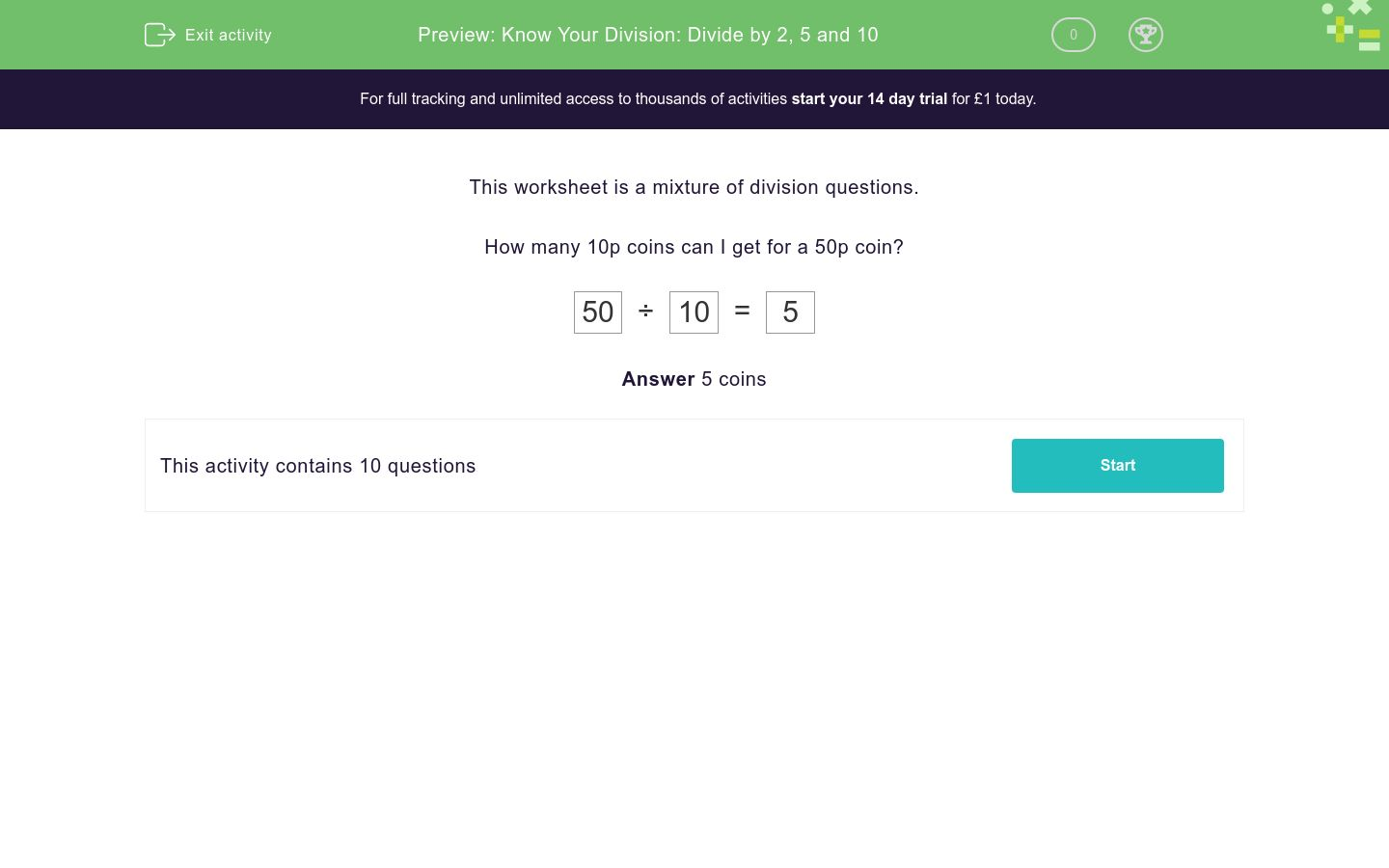# Know Your Division: Divide by 2, 5 and 10

In this worksheet, students answer a mixture of division questions.Key stage:  KS 1

Curriculum topic:   Number: Multiplication and Division

Curriculum subtopic:   Use Multiplication/Division Facts (2, 5, 10)

Difficulty level:### QUESTION 1 of 10

This worksheet is a mixture of division questions.

How many 10p coins can I get for a 50p coin?

 50 ÷ 10 = 5

How many 10 cm lengths of string can I cut from a 40 cm length?

 40 ÷ 10 = ?How many tens make 50?

 50 ÷ 10 = ?

A bird has 2 legs.

I see some birds and count 18 legs in total.

How many birds did I see?

 18 ÷ 2 = ?How many 2 p coins can I get for a 20 p coin?

 20 ÷ 2 = ?Share 12 between 2

 12 ÷ 2 = ?

Share 80 between 2.

 80 ÷ 2 = ?

How many 10 cm lengths of string can I cut from a 70 cm length?Share 90 between 2.

Divide 28 by 2.

How many 5 cm lengths of string can I cut from a 40 cm length?• Question 1

How many 10 cm lengths of string can I cut from a 40 cm length?

 40 ÷ 10 = ?4
EDDIE SAYS

40 ÷ 10 = 4

10 x 4 = 40

• Question 2

How many tens make 50?

 50 ÷ 10 = ?
5
EDDIE SAYS

50 ÷ 10 = 5

10 x 5 = 50

• Question 3

A bird has 2 legs.

I see some birds and count 18 legs in total.

How many birds did I see?

 18 ÷ 2 = ?9
EDDIE SAYS

18 ÷ 2 = 9

2 x 9 = 18

• Question 4

How many 2 p coins can I get for a 20 p coin?

 20 ÷ 2 = ?10
EDDIE SAYS

20 ÷ 2 = 10

10 x 2 = 20

• Question 5

Share 12 between 2

 12 ÷ 2 = ?
6
EDDIE SAYS

12 ÷ 2 = 6

2 x 6 = 12

• Question 6

Share 80 between 2.

 80 ÷ 2 = ?
40
EDDIE SAYS

80 ÷ 2 = 40

2 x 40 = 80

• Question 7

How many 10 cm lengths of string can I cut from a 70 cm length?7
EDDIE SAYS

70 ÷ 10 = 7

10 x 7 = 70

• Question 8

Share 90 between 2.

45
EDDIE SAYS

90 ÷ 2 = 45

2 x 45 = 90

• Question 9

Divide 28 by 2.

14
EDDIE SAYS

28 ÷ 2 = 14

2 x 14 = 28

• Question 10

How many 5 cm lengths of string can I cut from a 40 cm length?8
EDDIE SAYS

40 ÷ 5 = 8

8 x 5 = 40

---- OR ----

Sign up for a £1 trial so you can track and measure your child's progress on this activity.

### What is EdPlace?

We're your National Curriculum aligned online education content provider helping each child succeed in English, maths and science from year 1 to GCSE. With an EdPlace account you’ll be able to track and measure progress, helping each child achieve their best. We build confidence and attainment by personalising each child’s learning at a level that suits them.

Get started